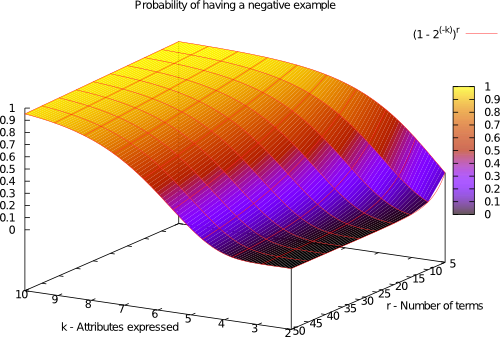# k-Disjunctive Normal Form functions

The k-Disjunctive Normal Form (k-DNF) functions are synthetic boolean functions that present different challenges for evolutionary learning in terms of number of attributes, size of the training set, overlapping and noise, among others.

In a space of d variables, the k-DNF functions have a following structure:

${T}_{1}\vee {T}_{2}\vee ...\vee {T}_{r}$

where r is the number of disjunctive terms and each term Tx represents the conjunction of k out of d variables (x1, x2, ..., xd). In boolean k-DNF functions a value of 0 or 1 is associated to each variable xi. The following is an example of k-DNF function in a space of 10 variables (d), with 3 terms/rules (r) using 4 variables/attributes (k) each.

$\left({x}_{1}\wedge {x}_{5}\wedge ¬{x}_{7}\wedge {x}_{8}\right)\vee \left({x}_{1}\wedge ¬{x}_{3}\wedge ¬{x}_{6}\wedge {x}_{7}\right)\vee \left({x}_{1}\wedge {x}_{2}\wedge ¬{x}_{6}\wedge {x}_{8}\right)$

The difficulty of learning a k-DNF function depends on the class imbalance and rule overlapping. The probability of having a negative example follows the following distribution:## k-DNF Generator

You can download the C++ source code of a k-DNF problem generator. The generator's input are the d, k, r parameters, the amount of noise and a random seed (to be able to reproduce the same sets). Instead of a complete k-DNF problem, the generator can produce just a random sample, which is useful for problems with a large amount of attributes and instances.

## Datasets

Two different k-DNF datasets have been used in our experiments:

The set includes 990 problems, 10 for each configuration with the parameters: d = , k = [2,3,4,5,6,7,8,9,10], r = [1,5,10,15,20,25,30,35,40,45,50]. These datasets have been used in Franco2010a.

The set includes 1800 problems, 5 for each configuration with the parameters: d = [10,20], k = [2,3,4,5,6,7,8,9,10], r = [1,5,10,20,40].
Each problem contains a certain ratio n of noise, where n = [0, 0.01%, 0.05%, 0.10%].

For any comments or questions about k-DNF problems or datasets in this website please send an e-mail to jaume.bacardit.

## Publications

• DOI slides BibTeX
M.A. Franco, N. Krasnogor, J. Bacardit
Analysing bioHEL using challenging boolean functions
in Proceedings of the 12th annual conference comp on Genetic and evolutionary computation - GECCO '10, p.1855, Portland, Oregon, USA, 2010
```@INPROCEEDINGS{Franco2010a,
title = {Analysing bioHEL using challenging boolean functions},
author = {Franco, Maria A. and Krasnogor, Natalio and Bacardit, Jaume},
year = 2010,
doi = {10.1145/1830761.1830817},
booktitle = {Proceedings of the 12th annual conference comp on Genetic and evolutionary computation - GECCO '10},
pages = {1855},